## Falling p.13

### Falling in 2 Dimensions: z Solutions

Our z solutions are exactly as in the 1-d fall. Thus it may be useful to recall what was said about the 1-d fall on page 7

Our normalized energy eigenfunctions are displaced Airy functions:These eigenfunctions are orthogonal: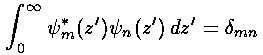So if we try to express a given function f(z') in terms of a sum of these functions:orthogonality allows us to solve for the bn:To see how this probability lump moves with time, we need only use the time dependence of the energy eigenfunctions:The only change from the 1-d fall is the initial condition. Before we were dealing with a particle dropped from rest. Here we seek a solution that moves upwards, reaches a maximum height, and then falls; that is we seek a solution with an upwards initial velocity. The previous pages have given us a model for the initial wavefunction of a moving wave-packet:= N exp(-y'2/42) exp(i k0y')

We need to modify it only a bit to use it for the initial state of our z motion. First, since U=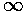for z<0,=0 for z<0. The gaussian wave=packet is never actually zero, just very small severals away from the peak. Thus if we continue to use a gaussian form, we must raise its CM location by several. Thus we limit the discontinuity at z=0 by makingsmall for positive z near 0 (whereas=0 for z<0). Thus we are led to an initial wavefunction:

f(z) = exp(-(z-z0)2/42) exp(i kz0z)

where z0/>> 1, so f(0+) is small. As before we must choserelatively large if we want a narrow range of velocities in the wave-packet. We then proceed to calculate the bn exactly as above. I chose kz0=12,=5, z0=20. I cannot actually find and infinite number of bn, so I restrict my sum about 150 n values whose eigenenergies are near the expected value of:

kz02 + z0 = 164

which occurs near n=450. Since I have not included the complete set of orthonormal wavefunctions in the sum, my solution to Schrödinger's equation will not exactly equal the gaussian form. (Furthermore I did not normalize my above f(z).) Hence I will want to normalize my finite-sum solution:Thus we have found our solution to the time dependent Schrödinger's equation for motion in the z direction which is a particular a superposition of single-energy solutions making a lump of probability that moves.

Here are some pictures of the probability density as it moves first up and then down:

### t=0### t=3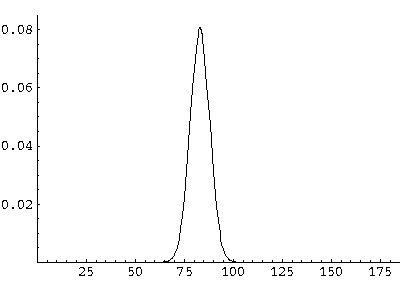### t=6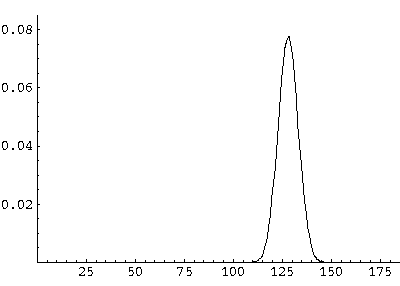### t=9### t=12### t=15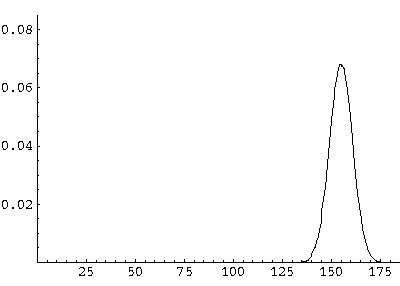### t=18### t=21### t=24All of these features can be seen in greater detail by examining the following QuickTime movie (0.2 Mb) on a frame-by-frame basis.